## Table of ContentOpen Access

ARTICLE

# The Full Load Voltage Compensation Strategy in Capacity Configuration of UPQC Integrated PV-BESS

Fuyin Ni1,2, Kai Li2,*

1 Jiangsu Key Laboratory of Power Transmission & Distribution Equipment Technology, Jiangsu University of Technology, Changzhou, 213001, China
2 School of Electrical and Information Engineering, Jiangsu University of Technology, Changzhou, 213001, China

* Corresponding Author: Kai Li. Email:Energy Engineering 2023, 120(5), 1203-1221. https://doi.org/10.32604/ee.2023.025796

## Abstract

Unified power quality conditioner (UPQC) with energy storage is commonly based on conventional capacity configuration strategy with power angle control. It has problems such as phase jumping before and after compensation. DC-link cannot continuously emit active power externally. Therefore, this paper presents the compensation strategy of full load voltage magnitude and phase in capacity configuration of UPQC. The topology of UPQC is integrated a series active power filter (SAPF), a shunt active power filter (PAPF) and a photovoltaic-battery energy storage system (PV-BESS). The principle of full load voltage compensation is analyzed based on the PV-BESS-UPQC topology. The magnitude constant of load voltage is maintained by controlling the appropriate shunt compensation current. Then the UPQC capacity configuration is carried out using the full load voltage compensation strategy. The compensation capacity of UPQC series and shunt units are reduced. Finally, the simulation results show that the proposed compensation strategy reduces the capacity configuration by 5.11 kVA (36.4%) compared to the conventional compensation strategy. The proposed strategy can achieve full compensation of the load voltage, which can effectively reduce the capacity allocation and improve the economy of UPQC. It also has the PV-BESS units' ability of providing active power and can stabilize the DC-link voltage.

## Graphical Abstract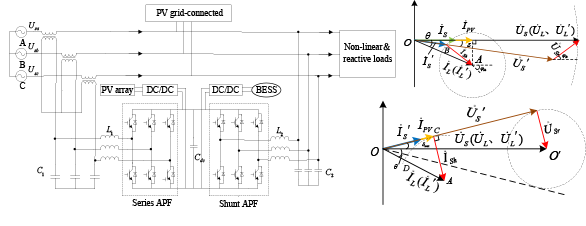## Keywords

1  Introduction

With the continuous development of the electric power industry, the power quality issue is getting more and more attention. Compared with the traditional electrical loads, the new generation of power distribution system consisting of power electronic devices also requires higher and higher power quality. Power quality becomes a problem that cannot be ignored [1,2]. Therefore, various power quality conditioners have emerged, such as active power filter (APF), static reactive power compensator (SVC) and dynamic voltage restorer (DVR). However, these conditioners can only solve part of the power quality problems. While the unified power quality conditioner (UPQC) can solve most of the power quality problems, so it has been discussed and studied by scholars .

For the UPQC-related compensation strategy with energy storage units on the DC-link, Devassy et al.  proposed a UPQC power compensation strategy based on power angle control. The series units emit a certain size of reactive power to share the capacity burden of the shunt units. But the power angle is not necessarily equal to the phase jump angle of the grid voltage. It may cause a phase jump before and after the load voltage compensation. In , a UPQC voltage compensation strategy based on reactive power control is proposed. It eliminates the active circulating current. But it presupposes that the compensation voltage on the series-connected unit should follow the principle of mutual perpendicularity with the supply current. That makes the phase of the compensated load voltage uncontrolled. It causes the phase jump of the load voltage before and after compensation. In , authors proposed a compensation strategy based on coordinated active and reactive power distribution. It maintains a constant compensation capacity of the shunt unit to distribute the active power provided by the power supply and the energy storage unit. But the voltage and current amplitudes on the series unit after compensation are large. That results in maintaining a high compensation capacity of the series unit. In , a UPQC power coordination compensation strategy where the load reactive power is mainly provided by the shunt unit is studied. When the load reactive power demand is greater than the rated capacity set by the shunt unit. The excess is provided by the series unit. But the capacity burden on the series unit is increased when the excess is larger.

It is thus clear that how to achieve full compensation of load voltage magnitude and phase. While reducing the capacity configuration and cost of UPQC is an urgent problem in current UPQC applications. Therefore, this paper takes the PV-BESS-UPQC as a topology of study. It analyzes the problems of conventional PV-BESS-UPQC power angle control. Then the compensation strategy of full load voltage magnitude and phase in capacity configuration has been proposed. Also, the capacity configuration principles and methods of series and parallel units are analyzed. Finally, the correctness and effectiveness of the proposed strategy are verified by simulation and experiment.

2  Conventional Power Angle Compensation Strategy in PV-BESS-UPQC

The structure of UPQC mainly consists of a series active power filter (SAPF) and a shunt active power filter (PAPF). The PV-BESS integrated UPQC is added with Boost converter and photovoltaic array. The construction of PV-BESS-UPQC is displayed in Fig. 1.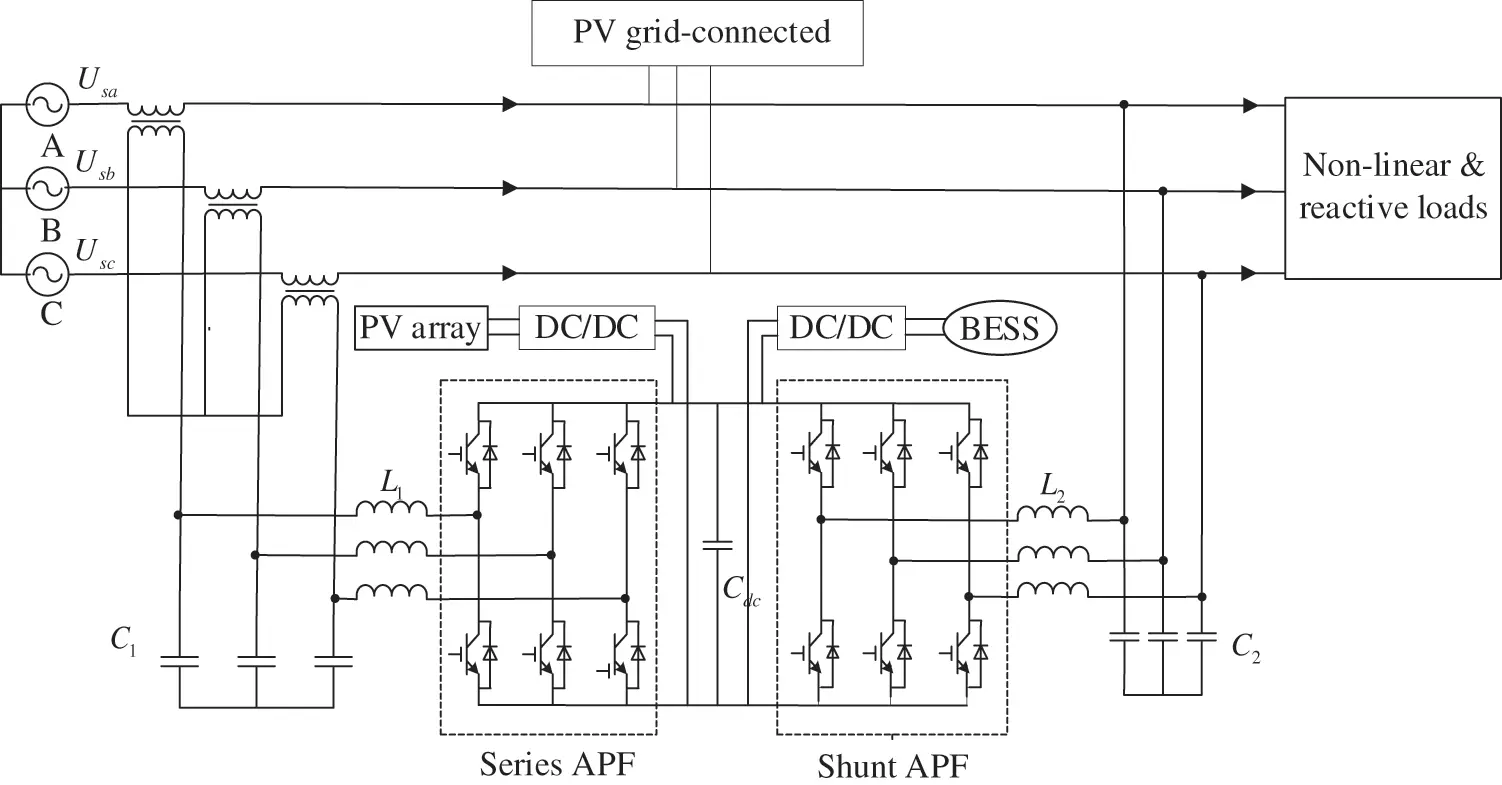Figure 1: System configuration of PV-BESS-UPQC

In conventional PV integrated UPQC compensation control, the series converter unit only operates when voltage drops occur and only provides active power and does not participate in the compensation of load reactive power. In , a UPQC-PV power compensation strategy based on power angle control is proposed. It makes the series converter unit emit a certain size of reactive power to share the burden of the shunt converter.

The phase diagram of UPQC-PV operation based on power angle control under voltage drops is displayed in Fig. 2. The core of UPQC power angle control is how to select the power angle δ, which can be changed by selecting different compensation voltage vectors U˙Sr. While the power angle δ also changes with the compensation angle U˙Sr. Selecting different angles δ can adjust the size of the series converter output power. Thus, the coordinated power distribution can be achieved when compensating the voltage. It is ensured that the series converter assures the load rating of U˙L after compensation. The selection of the shunt compensation current I˙Sh becomes more flexible due to the existence of PV energy storage unit. However, in order to keep the series unit always at rated capacity, this compensation strategy specifies that the rated operating voltage of the series unit Urat is equal to the amplitude of U˙S. The sum of the amplitudes of I˙S and I˙PV is equal to the active component of I˙L, so that the series unit still maintains a high capacity burden. U˙L only achieves amplitude compensation, but the phase jumps with U˙S. Consequently, the load voltage will always have phase jumps under this compensation strategy, which is not suitable for loads sensitive to phase.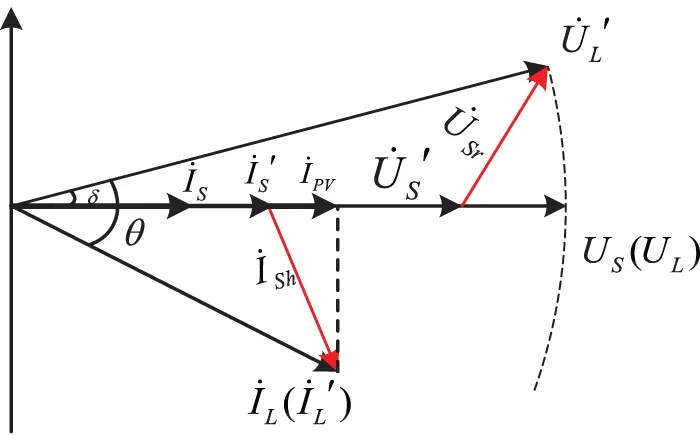Figure 2: UPQC-PV phase diagram based on power angle control

3  Strategy of Full Load Voltage Compensation in PV-BESS-UPQC

The magnitude and phase of voltage jump is the most common and the most concerned power quality problem for power users. In response to the problem of load voltage phase which is often not considered in the conventional energy storage UPQC power angle compensation strategy. This paper proposes a full load voltage compensation strategy of magnitude and phase. Its compensation phase diagram is shown in Fig. 3.Figure 3: Phasor diagrams for full compensation of load voltage

In summary, the full compensation of the load voltage considers the following four factors: The post-fault supply voltage U˙S is in phase with the compensated supply current I˙S, when the power factor angle of the network side θ=0. The amplitude of the compensated load voltage U˙L is equal to the amplitude of the load voltage U˙L during normal operation of the system, when the amplitude difference ΔU=0. The phase of the compensated load voltage U˙L is equal to the phase of the load voltage U˙L during normal operation of the system, when the phase difference Δδ=0. The active power conservation between the power supply, compensation device and load are conserved, when ΔP=0. The above four factors are also the four constraints of the UPQC voltage compensation strategy, which are expressed in the (1) by the mathematical formula:

{θ=|θUθI|=0ΔU=||U˙L||U˙L||=0Δδ=|δδ|=0ΔP=|PSPPVPL|=0(1)

In Eq. (1), θU and θI indicate the phase angle of supply voltage and voltage and current, respectively. |U˙L| and |U˙L| indicate the amplitude of load voltage before and after compensation, respectively. δ and δ indicate the phase angle of load voltage before and after compensation, respectively. PS, PPV and PL indicate the active power of supply, UPQC DC-link PV energy storage and load, respectively.

4  Capacity Configuration of PV-BESS-UPQC Based on Full Load Voltage Compensation Strategy

4.1 Selection of Compensation Current for UPQC Shunt Units

In the full load voltage compensation strategy, the magnitude of the supply current I˙S needs to be minimized in order to keep the compensation capacity of the series unit low. Since the magnitude of the load voltage remains constant before and after compensation. I˙S is affected by the compensation current of the shunt unit I˙Sh. Therefore, it becomes essential to choose the magnitude of I˙Sh to reduce the capacity configuration of UPQC.

Specifically, the appropriate shunt compensation current I˙Sh should be selected firstly. Its magnitude remains constant. As shown in Fig. 4, the end point A of I˙L is the center of the circle, the amplitude of I˙Sh is the radius of the circle. The intersection of circle A and U˙S is B. In order to ensure that the power factor of the compensated power supply is 1, it is necessary to keep the compensated power supply current I˙S in phase with the power supply voltage U˙S. OB is selected as the compensated power supply current I˙S.Figure 4: Phase diagram of I˙sh amplitude selection

As shown in Fig. 4 above, the phase diagram of I˙Sh amplitude selection is shown. The amplitude I˙Sh selection method is as follows: In order to make the network power factor 1, it is ensured that the supply current I˙S is in phase with the supply voltage U˙S after the fault. When the phase jump angle δ of U˙S is equal to the maximum allowable jump angle δMAX (the variation range of δ is δMAXδδMAX), the load currents I˙L and I˙L remain unchanged before and after compensation. This paper makes a vertical line from point A to U˙S and intersect at point C. Then OC is the supply current I˙S and CA is the shunt unit compensation current I˙Sh. At this time, the vertical line CA is the shortest line segment from point A to U˙S. |CA| is the minimum shunt unit compensation current I˙Sh and the selected amplitude of I˙Sh. The point P which ends of I˙L is used as the center. |CA| is chosen as the radius of the circle. The arc CD is the trajectory of I˙Sh end point.

In Fig. 4, the center of the circle is chosen with O at the end of U˙S. |U˙Sr| is the radius of the circle. The line OA is its tangent. The magnitude of U˙Sr and the magnitude of U˙S are related as follows:

|U˙Sr||U˙S|=sinδmax(2)

In summary, U˙S varies within the circle O. |CA| is the selected as the amplitude of I˙Sh.

4.2 Capacity Configuration of Series Unit of UPQC

For the convenience of analysis, the supply voltage drop factor k in (3) is defined as the ratio of the magnitude of U˙S to the rated of U˙S after the drop.

k=|U˙s||U˙s|(3)

According to the compensation triangle composed of phase quantities U˙S, U˙S, and U˙Sr in Fig. 4, the amplitude and phase of U˙Sr is obtained from the trigonometric cosine theorem as:

{|U˙Sr|=U1+k22kcosδφSr={arctanksinδ1kcosδ,kcosδ1180arctanksinδkcosδ1,kcosδ>1(4)

According to the compensation triangle formed by the phase quantities I˙S, I˙Sh, and I˙L in Fig. 4, the amplitude of I˙S is obtained from the trigonometric cosine theorem as follows:

|I˙S|+|I˙PV|=I(cos(θ+δ)sin2(θ+δmax)sin2(θ+δ))(5)

I˙PV is the current output from the PV array through the UPQC shunt cell. There exist the following relationships:

|I˙PV|=Icosθ|I˙S|=Icosθ|I˙S|cosδmax(6)

The phase angle δ of I˙S is equal to the phase jump angle of U˙S. When δ=δmax, it is easy to see from Fig. 4 that the magnitude of I˙S reaches its maximum value. Its magnitude is expressed as follows:

|I˙S|max=I[(1+1cosδmax)cos(θ+δmax)cosθ](7)

The compensation capacity of the series unit is expressed as:

SSr=|U˙Sr||I˙S|max=sinδmaxUI[(1+1cosδmax)cos(θ+δmax)cosθ](8)

Normally to meet the requirements of high power factor, power factor is generally required to be 0.85 to 0.9. The variation range of θ is: θ(25,32). The Fig. 5 shows the three-dimensional trend of the SSr with the allowable jump angle δ and the load power factor angle θ. From the Fig. 5, it can be seen that the compensation capacity of the series unit reaches the maximum when θ=25.84 and δmax=22. The rated capacity of the series unit is designed as:

SSrmax=0.19UI(9)Figure 5: Three-dimensional relationship between compensation capacity of series unit

In this conventional compensation strategy, it is stipulated that the amplitude of U˙Sr is equal to the rated working voltage of the supply voltage. Since the load power factor θ is greater than 0.9, reactive power compensation is not required. The rated capacity of the series unit of this conventional compensation strategy is designed as follows:

SSrmax=0.9UI(10)

From Eqs. (9) and (10), it can be seen that the installed capacity required for the series-connected units of the compensation strategy proposed can be reduced 0.71UI compared to that of the conventional compensation strategy.

4.3 Capacity Configuration of Shunt Unit of UPQC

In Fig. 3, U˙S is equal to U˙L during normal system operation. After a supply voltage drop, I˙L is equal to I˙L. U˙L is equal to U˙L. The relationship is shown in (11).

{|U˙S|=|U˙L|=|U˙L|=U|I˙L|=|I˙L|=I(11)

From the selection method of I˙Sh, it can be seen that the amplitude of CAOC in Fig. 4. The amplitude of I˙Sh is equal to the amplitude of CA. It is easy to find the amplitude in ΔOAC is expressed as:

|I˙Sh|=Isin(θ+δmax)(12)

According to the compensation triangle formed by the phase quantities I˙S, I˙Sh, I˙L in Fig. 3, it is obtained from the trigonometric cosine theorem that the relationship is expressed as:

|I˙L|sinOBA=|I˙Sh|sin(θδ)(13)

From the Eq. (13), OBA is derived as:

OBA=90+arcsin(sin(θδ)sin(θ+δmax))(14)

Further from the trigonometric relationship, the phase angle of I˙Sh is derived as:

φSh=180OBA+δ=90arcsin(sin(θδ)sin(θ+δmax))+δ(15)

From the above analysis, it can be seen that the compensation capacity of the shunt unit under the compensation strategy proposed in this paper is shown as follows:

SSh=|U˙L||I˙Sh|=UIsin(θ+δmax)(16)

The relationship between the shunt unit compensation capacity SSh, the power factor angle θ and the maximum phase jump angle δmax is shown in Eq. (16). When θ+δmax=90, the shunt unit compensation capacity reaches the maximum. The rated compensation capacity of the shunt unit is designed as:

SSh,max=UI(17)

In the conventional power angle compensation strategy shown in Fig. 2, the shunt compensation capacity in the event of a supply voltage drop is obtained as:

SSh=UI1+cos2θsin2θ(18)

And the power factor is generally required to be 0.85 to 0.9. The variation range of θ is: θ(25.84,32.79). When θ=25.84, the shunt unit compensation capacity reaches the maximum, so the rated capacity of the shunt unit of this conventional compensation strategy is shown as follows:

SSh,max=UI(19)

From Eqs. (17) and (19), it can be seen that conventional power angle strategy requires the same installed capacity as the shunt units of the compensation strategy proposed in this paper.

4.4 Capacity Configuration of PV-BESS Unit of UPQC

Configuration of PV-BESS unit capacity includes the capacity configuration of PV arrays and the energy storage system. The capacity configuration in PV power generation output is mainly affected by solar radiation illumination, ambient temperature and other factors. The capacity of PV arrays is designed as follows:

EPV=APVGηPVηinv(20)

where: APV is the area of PV panel receiving solar radiation. G is the value of light radiation. ηPV is the energy conversion efficiency of PV unit. ηinv is the conversion efficiency of DC/DC converter of PV unit. The energy conversion efficiency is also related to the temperature of the environment.

The capacity of the energy storage system is mainly determined by the following parameters: the size of the active power of the load, the degree of drop of the supply voltage and the duration of the drop. The capacity is determined by the worst possible working conditions of the user load. The worst working condition of the load considers the situation of power interruption. When the energy storage unit realizes the uninterrupted power supply to the load, that is, the load active power is completely provided by the energy storage unit. The capacity of the energy storage unit is designed as follows:

where: Pload is the active power of the load. Udc is the DC-link voltage. t is the duration of uninterrupted power supply. λ is the rated capacity margin.

In summary, the compensation strategy proposed in this paper achieves complete compensation of the load voltage. Compared with the conventional compensation strategy, the compensation capacity of UPQC can be greatly reduced. The capacity cost of UPQC series and shunt units can be saved. Also, the capacity design of PV-BESS unit ensures the uninterrupted power supply to the load.

5  Control Strategy of PV-BESS-UPQC

5.1 Control Strategy of Series-Shunt Unit in UPQC

The Figs. 6 and 7 show the diagram of series and shunt units control strategy of UPQC. In these figures, L1 and L2 are the series and shunt unit outlet filter inductors, respectively. Fig. 6 shows the series converter control strategy unit. usrabc is the reference value of series converter compensation voltage in the three-phase coordinate system derived from Eq. (4). usrabc is transformed by Park to derive the compensation voltages usrd, usrq for the dq coordinate system. usrabc is the actual value of series converter compensation voltage in the three-phase coordinate system. usrabc is transformed by Park to derive the compensation voltages usrd, usrq for the dq coordinate system. The difference between usrd, usrq and usrd, usrq is controlled by PI to eliminate the steady-state error and to realize the series voltage compensation function. usrd and usrq can be added to the control link as feed-forward compensation to increase the speed of the PI controller.Figure 6: Control strategy of UPQC series unitFigure 7: Control strategy of UPQC series unit

To achieve compensation of possible harmonic components in the supply voltage, the required voltage harmonic compensation value is extracted by a low-pass filter. This harmonic compensation value is used as part of the UPQCsr reference voltage signal. PI control is used to decouple the p-axis and q-axis for the error signal between the reference and actual value.

The control block diagram of the shunt converter unit is shown in Fig. 7. Based on the full compensation strategy, UPQCsh reference current signals isha, ishb and ishc are transformed to obtain the reference current signals ishd and ishq in the dq coordinate system. In order to achieve the compensation of possible harmonic components in the load current, the required harmonic compensation value of the load-side current is extracted by a low-pass filter. This harmonic compensation value is used as a component of the UPQCsh reference current signal to meet the requirements for the current waveform. PI control is used to decouple the p-axis and q-axis for the error signal between the reference signal and the actual value to complete the current tracking control compensation of UPQCsh. In addition, the UPQCsh voltage feed-forward compensation is also added to the UPQCsh control in order to achieve fast switching and response of the device.

5.2 Control Strategy of PV-BESS Unit in UPQC

The PV-BESS configuration displayed in Fig. 8 comprises the PV array, BESS, Boost converter, Buck/Boost converters. The BESS is attached parallelly to the DC-link capacitor utilizing buck-boost converter, so the stability of the UPQC is improved for compensating power quality problem. The BESS unit is charged and discharged through a bidirectional Buck/Boost converter connected to the DC-link of the UPQC. It is in Buck circuit mode when T2 is disconnected and T3 is on, the power supply charges the energy storage unit. It is in Boost circuit mode when T3 is disconnected and T2 is on, the PV-BESS unit releases excess energy to meet the requirements of the system load. Under normal operating conditions, the output current ISh of the UPQC shunt converter can be changed to increase the power supply current IS, so that the power supply can generate more active power to charge the BESS unit.Figure 8: PV array and bidirectional Boost converter structural diagram (a), BESS and bidirectional Buck/Boost converter structural diagram (b)

When the grid voltage drop is deep, the PV-BESS unit can play a limiting role on the grid current. It can reduce the risk of insufficient compensation capacity of UPQC in the case of deep grid voltage drop. In order to meet the fast energy leveling and improve the DC-link voltage constancy, the DC-link adopts the double closed-loop control strategy of voltage external loop control and current internal loop control. It enhances the ability of bus voltage self-regulation.

The PV system output power, PPV is boosted by the DC-DC Boost converter that control by controller shows in Fig. 9. The controller of the DC-DC Boost converter is operating by getting DC voltage error, uDCerror. The voltage error is calculated by comparing the given reference voltage, uref which is 800 V. The reference voltage is assigned with instantaneous DC-DC Boost converter output DC voltage uDC. However, the controller of the DC-DC Boost converter will break off the PV system output power, PPV during battery’s state of charge SOCBESS is over or equal to 98% of battery capacity. The reason is to keep away the battery from being overcharged and unstable. Moreover, the lifetime of the battery can be reduced due to overcharging.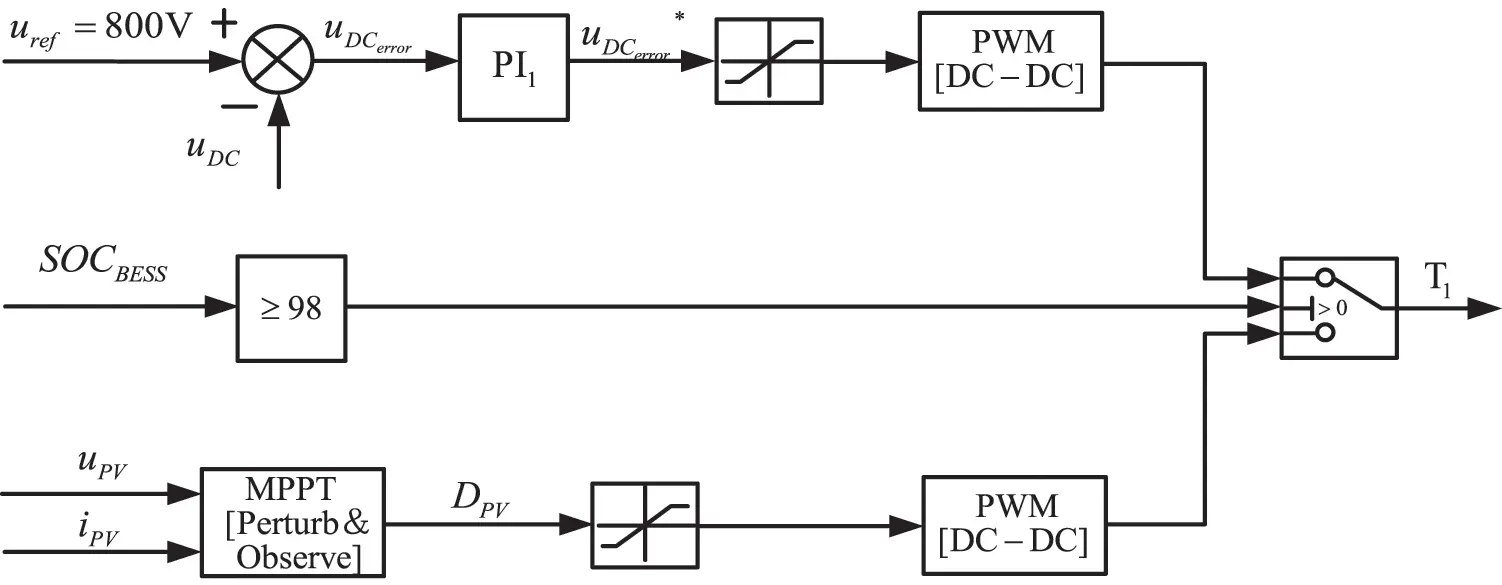Figure 9: BESS DC-DC buck-boost converter controller scheme

In Fig. 10, it shows the bidirectional converter Buck-Boost DC-DC controller for charging and discharging mode that contains embedded internal control loops and external control loops. The difference between the DC voltage reference value uref and the actual output DC voltage uDC is generated by the PI controller to generate the internal loop current reference value iBESSref. The difference between the iBESSref and the actual current iBESS is realized by the PI controller to generate the modulation signal of the DC/DC converter without difference control. It finally achieves the purpose of stabilizing the DC voltage and regulating the power output.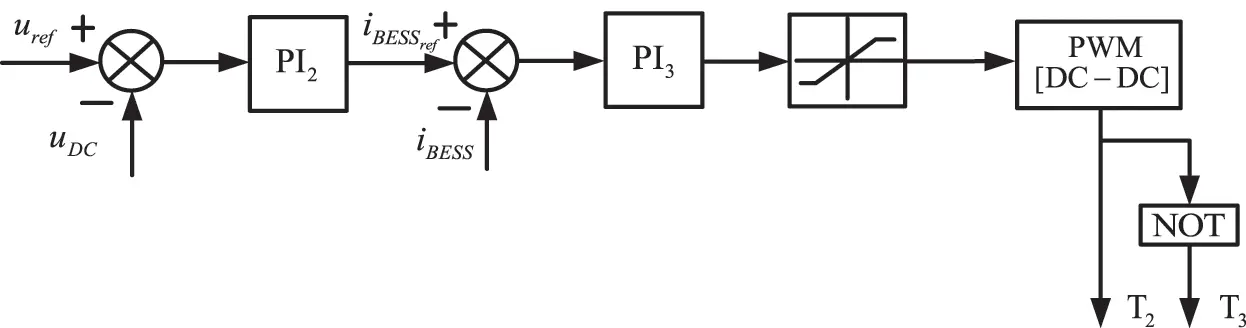Figure 10: BESS DC-DC buck-boost converter controller scheme

6  Simulation Results

In order to verify the correctness and effectiveness of the proposed full load voltage compensation strategy in capacity configuration of UPQC integrated PV-BESS. The series and shunt unit compensation capacities are simulated using the MATLAB/Simulink simulation platform. The conventional compensation strategy and the full load voltage compensation strategy proposed in this paper are compared, respectively. The UPQC-PV related simulation parameters are set in Table 1.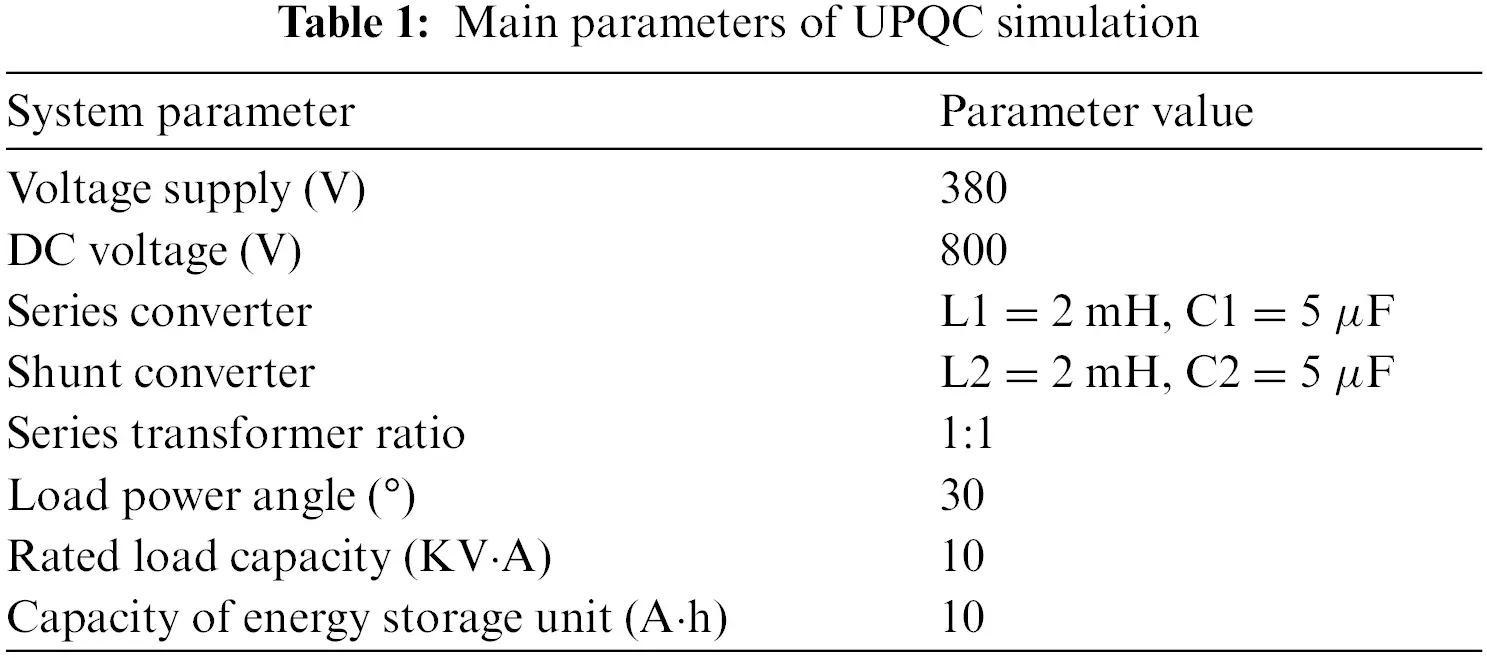6.1 Case Study 1

The simulation is set at 2 s when the phase of the power supply voltage has a transient 20% drop of amplitude (60 V) and a δ=15 phase jumping fault. Respectively, the conventional PV-BESS-UPQC power angle compensation strategy and the full load voltage compensation strategy proposed in this paper are compared. Load voltage phase jumping is verified by simulation. The obtained voltage and current simulation results are shown in Fig. 11.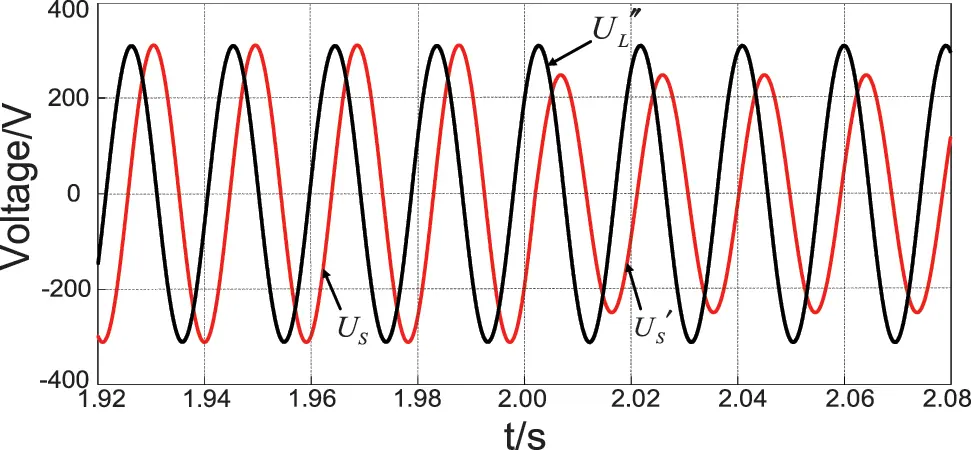Figure 11: Conventional power angle compensation strategy supply voltage, load voltage waveform

The comparison of the supply voltage and load voltage waveforms before and after using the conventional power angle compensation strategy is shown in Fig. 11. Before 2 s in the simulation, when the supply voltage works normally, the load voltage UL is compensated by the series unit compensation voltage vector USr. The amplitude of UL is equal to the amplitude of US. However, the phase jumps with respect to the phase of US. After 2 s in the simulation, when the supply voltage drops. The load voltage only achieves the amplitude compensation but not the phase compensation after the series unit compensates the voltage vector. The load voltage always has a phase jump.

When using the full load voltage compensation strategy proposed in this paper. The comparison of supply voltage and load voltage waveforms before and after compensation is shown in Fig. 12a. Before 2 s in the simulation, the amplitude and phase of load voltage UL are equal to supply voltage US after USr compensation when the supply voltage is working normally. The amplitude and phase of remains unchanged after compensation when the supply voltage drops 2 s later. After 2 s in the simulation, the amplitude and phase remain the same after compensation. The load voltage is fully compensated.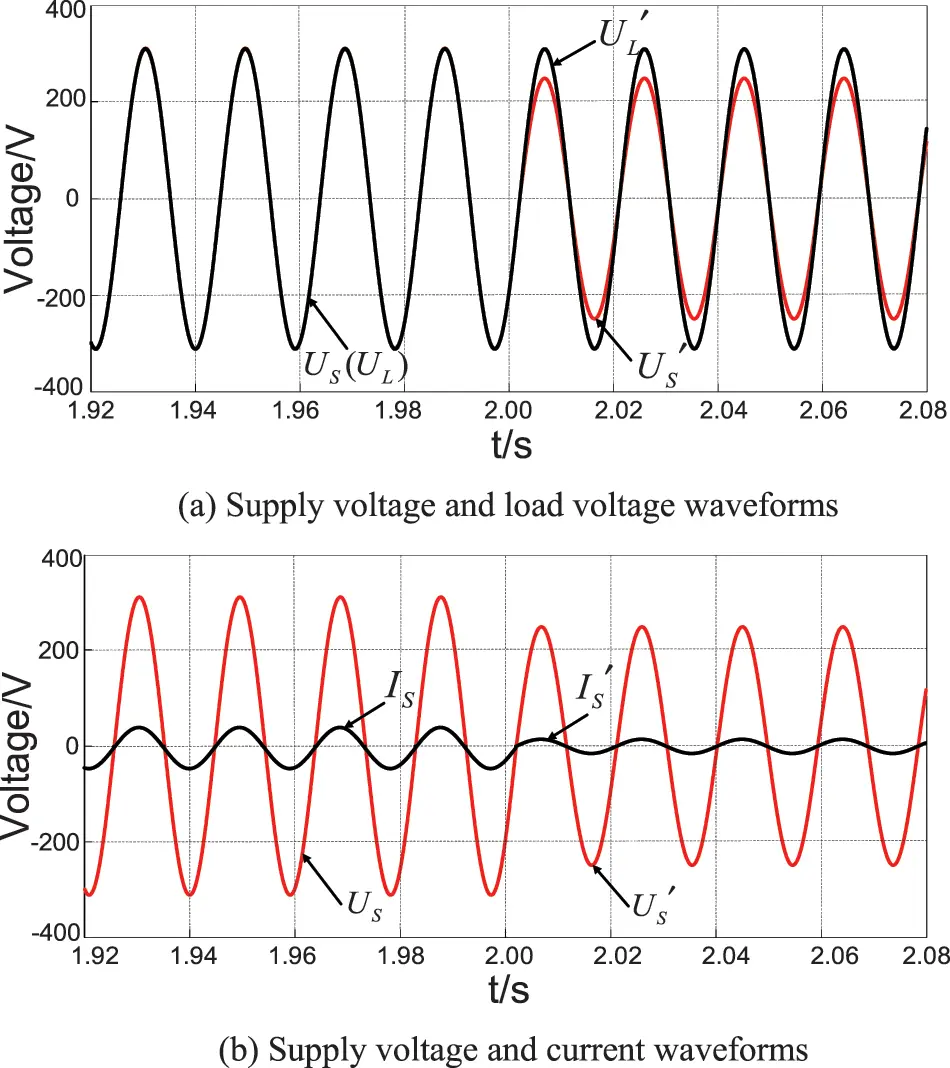Figure 12: The proposed full load voltage compensation strategy supply voltage and load voltage waveforms (a), supply voltage and current waveforms (b)

The waveforms of supply voltage and load voltage before and after compensation are shown in Fig. 12b. When the supply voltage works normally, before 2 s in the simulation, the supply current IS is in phase with the supply voltage US after compensation by the shunt unit compensation current vector ISh. After 2 s in the simulation, when the supply voltage drops, IS and US are still in phase after compensation by ISh. The power factor is always 1 when the power supply before and after compensation.

When using the conventional power angle compensation strategy. The active and reactive power simulation waveforms of each unit of the system are shown in Fig. 13. When the supply voltage is working normally, the power provides all the active power 8.66 kW to the load. The reactive power provided by series unit is 7.50 kVar. The reactive power provided by shunt unit is 1.16 kVar. The series and shunt units provide all the reactive power 8.66 kVar to the load. After 1 s, when the power supply voltage drops, the active power provided by the power supply decreases to 6.93 kW. As shown in Figs. 13a and 13d, the difference of active power 1.73 kW is provided by the energy storage unit to the load through the shunt unit. Meanwhile, the active power absorbed by the series unit decreases to 3.34 kW. The reactive power provided by series unit increases to 7.99 kVar. The compensation capacity of 8.66 kVA after voltage drops is kept unchanged, as shown in Fig. 13b. The active power provided by the shunt unit increases to 5.34 kW. The reactive power provided by the shunt unit decreases to 0.65 kVar. The compensation capacity after voltage drops increases to 5.38 kVA, as shown in Fig. 13c. From the above simulation data, the total capacity provided by the system after the supply voltage drop is 14.04 kVA using the conventional compensation strategy.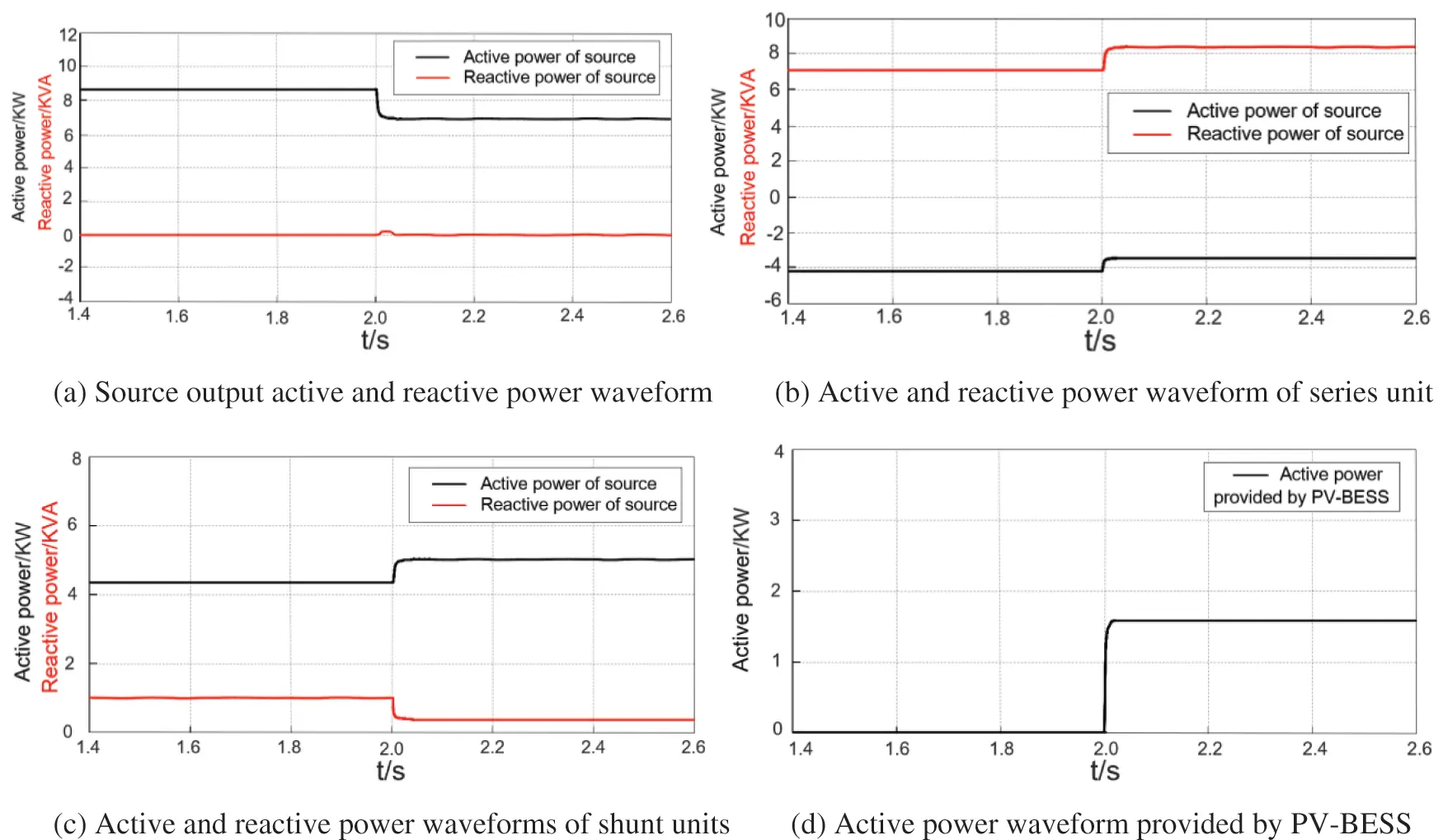Figure 13: Active and reactive power waveforms of conventional compensation strategy

The simulation results using the compensation strategy proposed in this paper are shown in Fig. 14. When the system works normally, the grid provides all the active power 8.66 kW. The shunt unit provides all the reactive power 8.66 kVar to the load. The active power provided by the power supply is reduced to 1.48 kW after 2 s. The difference of active power 7.18 kW is provided by the energy storage unit to the load through the series and shunt units, as shown in Figs. 14a and 14d. Meanwhile, the active power provided by the series unit increases to 0.80 kW. The reactive power provided by the series unit increases to 1.25 kVar. The compensation capacity increases to 1.48 kVA after a voltage drop, as shown in Fig. 14b. The active power provided by the shunt unit increases to 0.79 kW. The reactive power provided by the shunt unit decreases to 7.41 kVar. The compensation capacity increases to 7.45 kVA after voltage drops in Fig. 14c. From the above simulation data, the total capacity provided by the system is 8.93 kVA.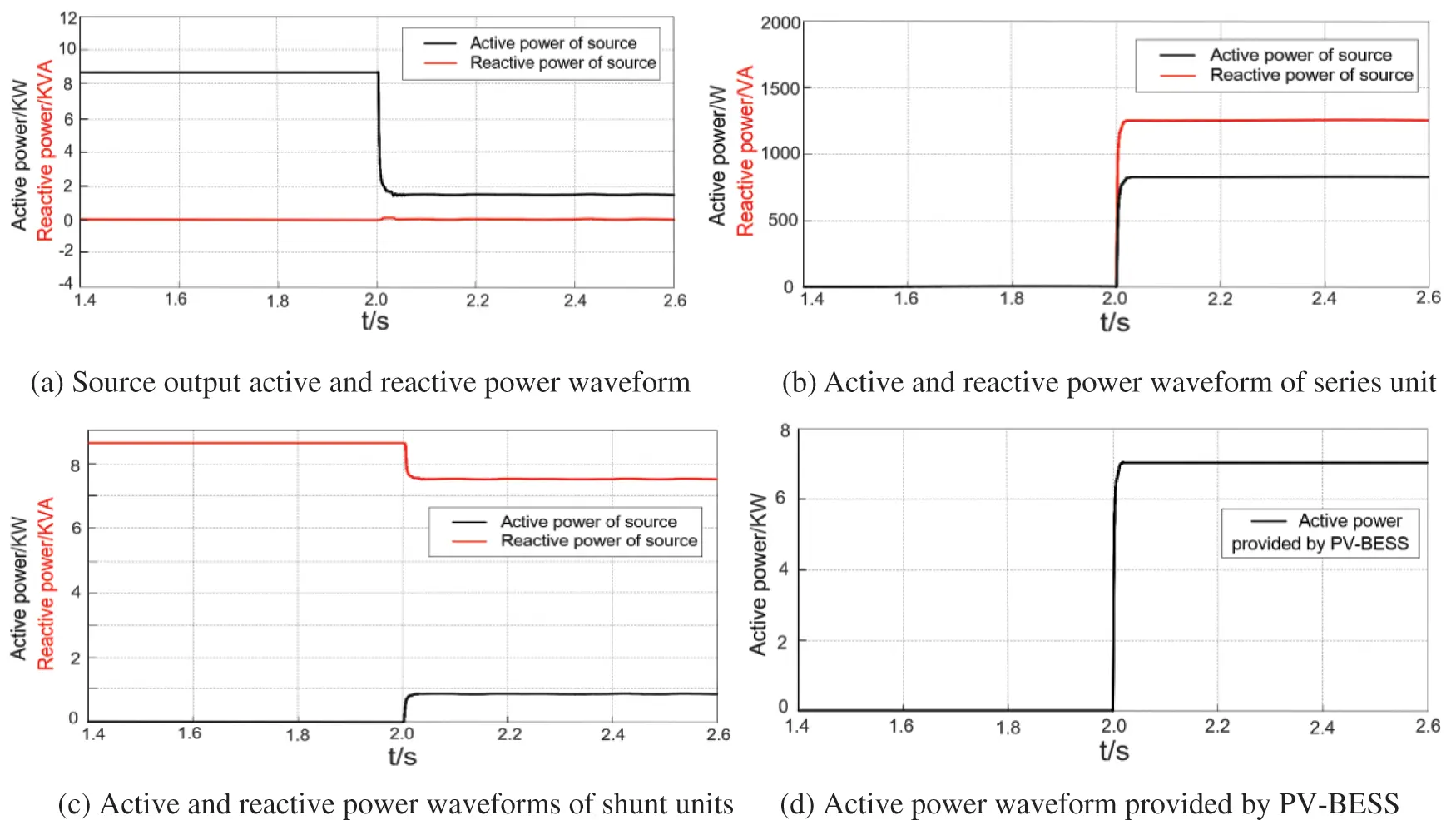Figure 14: Active and reactive power waveforms of proposed compensation strategy

6.2 Case Study 2

In order to further verify the function of UPQC with PV-BESS to supply continuous power to the load when the supply voltage drops completely under the proposed control strategy. The power supply is set to open circuit at 2 s. The system simulation results are shown in Figs. 15, 16a and 16b. As shown in Figs. 15, 16a and 16b, the series converter compensates the load voltage to the rated value with the amplitude of 310.26 V in the special case that the supply voltage drops to zero. It ensures the uninterrupted power supply to the load. Fig. 16b shows that the feeder current magnitude remains constant when the voltage drops completely. Figs. 17a17d show that when the supply voltage is normal, the power supply assumes the full active power of the load of 8.66 kW. The series converter and shunt converter together assume the reactive power of 8.66 kVA. When the extreme case of the supply voltage drop occurs, the PV-BESS unit provides the full active power of the load. At which time the power of the load reactive unit is distributed in a coordinated manner.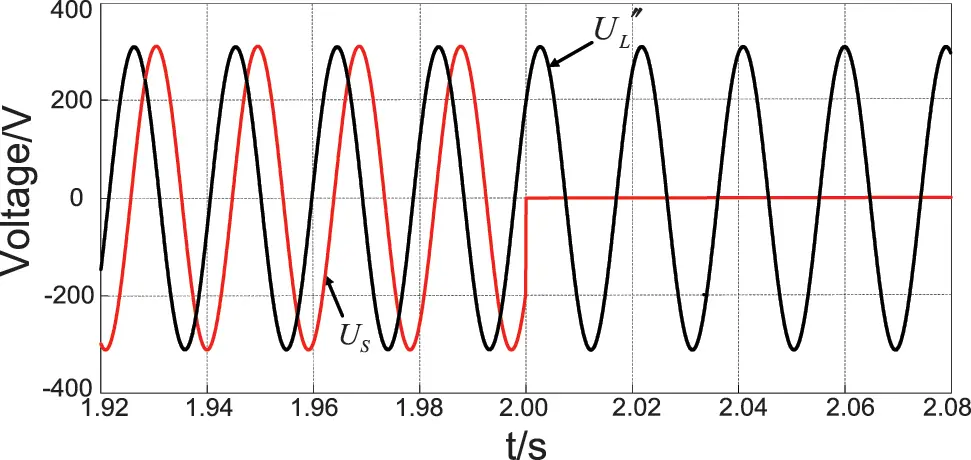Figure 15: Conventional power angle compensation strategy supply voltage, load voltage waveformFigure 16: The proposed full load voltage compensation strategy supply voltage and load voltage waveforms (a), supply voltage and current waveforms (b)Figure 17: Active and reactive power waveforms of proposed compensation strategy

In summary, when in the case of a fault with a 20% drop in power supply voltage and a jump in phase. The proposed compensation strategy increases the compensation capacity of shunt units by 2.07 kVA compared to the conventional compensation strategy. But it reduces the compensation capacity of series units by 7.18 kVA. That results in a reduction of 5.11 kVA (36.4%) in the total system capacity. Therefore, the proposed compensation strategy reduces the capacity allocation of UPQC by reducing the compensation capacity of series units. The series converter compensates the load voltage to the rated value with the amplitude of 310.26 V in the special case that the supply voltage drops to zero. It ensures the uninterrupted power supply to the load. The PV-BESS unit provides the full active power of the load. At which time the power of the load reactive unit is distributed in a coordinated manner.

7  Conclusion

In this paper, a full load voltage compensation strategy in capacity configuration is proposed for PV-BESS-UPQC. The problems of phase jump and high compensation capacity of series units after the compensation strategy are pointed out. Then, the compensation strategies proposed in this paper are analyzed. Through the comparative analysis of the two compensation strategies, the main conclusions are as follows:

1)   By reasonably distributing the power of UPQC series unit, shunt unit and PV-BESS unit, the capacity configuration of UPQC can be effectively reduced and the compensation performance of UPQC can be improved.

2)   The PV-BESS unit is used to maintain the power supply current as the active component of the load current under normal conditions. It reduces the feeder current increased when the power supply voltage drops and eliminates the risk of feeder overcurrent in the distribution network.

3)   In the special case that the power supply voltage drops to zero, the PV-BESS unit provides all the active power of the load at this time. It ensures the uninterrupted power supply to the load.

Funding Statement: Supported by Open Project of Jiangsu Key Laboratory of Power Transmission & Distribution Equipment Technology (2021JSSPD12) Supported by Talent Projects of Jiangsu University of Technology (KYY20018). Supported by Graduate Practice Innovation Program of Jiangsu University of Technology (XSJCX21_32).

Conflicts of Interest: The authors declare that they have no conflicts of interest to report regarding the present study.

## References

1. Tan, Z., Luo, L. F., Cui, G. P., Hong, W. C., & Tian, Y. (2020). UPQC with coupled controllable reactors. Electric Power Automation Equipment, 40(1), 101-105. [Google Scholar]
2. Huang, X. M., Fan, Z. H., Liu, Z. W., Miao, S., & Li, X. W. (2018). Control strategy of UPQC based on active and reactive power coordination distribution. Electric Power Automation Equipment, 38(3), 177-183. [Google Scholar]
3. Khadkikar, V. (2012). Enhancing electric power quality using UPQC: A comprehensive overview. IEEE Transactions on Power Electronics, 27(5), 2284-2297. [Google Scholar]
4. Khadem, S. K., Basu, M., & Conlon, M. F. (2015). Intelligent islanding and seamless reconnection technique for microgrid with UPQC. IEEE Journal of Emerging & Selected Topics in Power Electronics, 3(2), 483-492. [Google Scholar] [CrossRef]
5. Khadem, S. K., Basu, M., & Conlon, M. F. (2016). A comparative analysis of placement and control of UPQC in DG integrated grid connected network. Sustainable Energy, Grids and Networks, 6(1), 46-57. [Google Scholar] [CrossRef]
6. Devassy, S., Singh, B. (2017). Design and performance analysis of three-phase solar PV integrated UPQC. IEEE Transactions on Industry Applications, 54(1), 73–81.
7. Campanhol, L. B. G., da Silva, S. A. O., de Oliveira, A. A., & Bacon, V. D. (2019). Power flow and stability analyses of a multifunctional distributed generation system integrating a photovoltaic system with unified power quality conditioner. IEEE Transactions on Power Electronics, 34(7), 6241-6256. [Google Scholar] [CrossRef]
8. Lakshmi, S., & Ganguly, S. (2019). Multi-objective planning for the allocation of PV-BESS integrated open UPQC for peak load shaving of radial distribution networks. The Journal of Energy Storage, 22, 208-218. [Google Scholar] [CrossRef]
9. Goud, B. S., & Rao, B. L. (2021). Power quality enhancement in grid-connected PV/wind/battery using UPQC: Atom search optimization. Journal of Electrical Engineering & Technology, 16(2), 821-835. [Google Scholar] [CrossRef]
10. Karanki, G., Mishra, M. K., & Kumar, B. K. (2013). A modified three-phase four-wire UPQC topology with reduced DC-link voltage rating. IEEE Transactions on Industrial Electronics, 60(9), 3555-3566. [Google Scholar] [CrossRef]
11. Dash, S. K., & Ray, P. K. (2018). Novel PV-tied UPQC topology based on a new model reference control scheme and integral plus sliding mode DC-link controller. International Transactions on Electrical Energy Systems, 28(2), e2564. [Google Scholar] [CrossRef]
12. Reddy, P. M., Reddy, A. S., & Sarvesh, B. (2021). Hybrid ANFIS-FA-based control strategy for UPQC-power quality enhancement in smart grid. International Journal of Power Electronics, 13(4), 399-433. [Google Scholar] [CrossRef]
13. Devassy, S., & Singh, B. (2017). Modified pq-theory-based control of solar-PV-integrated UPQC-S. IEEE Transactions on Industry Applications, 53(5), 5031-5040. [Google Scholar] [CrossRef]
14. Lakshmi, S., & Ganguly, S. (2020). Multi-objective planning for the allocation of PV-BESS integrated open UPQC for peak load shaving of radial distribution networks. The Journal of Energy Storage, 22(2), 208-218. [Google Scholar] [CrossRef]
15. Singh, M. K., Kumar, A., & Gupta, A. R. (2022). Distribution system analysis with UPQC allocation considering voltage dependent time-variant and invariant loads including load growth scenario. Journal of the Institution of Engineers (India): Series B, 103(3), 791-807. [Google Scholar] [CrossRef]
16. Feng, X. T., Zhang, L. X., & Kang, Z. J. (2014). Working conditions and control strategy of UPQC based on supercapacitor energy storage. Electric Power Automation Equipment, 34(4), 84-89. [Google Scholar]
17. Patel, A., Mathur, H. D., & Bhanot, S. (2020). Enhancing VA sharing between the shunt and series APFs of UPQC with a modified SRF‐PAC method. IET Power Electronics, 13(2), 275-285. [Google Scholar] [CrossRef]
18. Patel, A., Yadav, S. K., Mathur, H. D., Bhanot, S., & Bansal, R. C. (2020). Optimum sizing of PV based UPQC-DG with improved power angle control. Electric Power Systems Research, 182(7), 106259. [Google Scholar] [CrossRef]

Ni, F., Li, K. (2023). The Full Load Voltage Compensation Strategy in Capacity Configuration of UPQC Integrated PV-BESS. Energy Engineering, 120(5), 1203–1221.This work is licensed under a Creative Commons Attribution 4.0 International License , which permits unrestricted use, distribution, and reproduction in any medium, provided the original work is properly cited.

View

Like

## Related articles

• Jian Ni, Mei Yang, Shengjie Zhai,...
• Shengqi Zhang, Bei Yuan, Jianfeng...
• D. Menaga, M. Premkumar, R. Sowmya,...
• Emad H. El-Zohri, Hegazy Rezk,...
• Ramkiran Bhallamudi, Sudhakar...